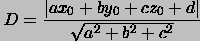## Equations of Lines and Planes

#### Lines in Three Dimensions

A line is determined by a point and a direction. Thus, to find an equation representing a line in three dimensions choose a point P_0 on the line and a non-zero vector v parallel to the line. Since any constant multiple of a vector still points in the same direction, it seems reasonable that a point on the line can be found be starting at the point P_0 on the line and following a constant multiple of the vector v (see the figure below).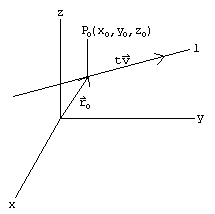If r_0 is the position vector of the point P_0, then the line must have the form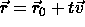This is the vector equation of a line in three dimensions. By letting r=<x,y,z>, r_0=<x_0,y_0,z_0>, and v=<a,b,c> we obtain the equation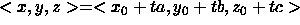which leads to the parametric equations of the line passing through the point P_0=(x_0,y_0,z_0) and parallel to the vector v=<a,b,c>: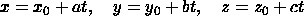#### Example

To find the parametric equations of the line passing through the point (-1,2,3) and parallel to the vector <3,0,-1>, we first find the vector equation of the line. Here, r_0=<-1,2,3> and v=<3,0,-1>. Thus, the line has vector equation r=<-1,2,3>+t<3,0,-1>. Hence, the parametric equations of the line are x=-1+3t, y=2, and z=3-t.

It is important to note that the equation of a line in three dimensions is not unique. Choosing a different point and a multiple of the vector will yield a different equation. If we had taken the point to be (2,2,-4) and the vector to be <-6,0,2> in the previous example we would have found the parametric equations of the line to be x=2-6t, y=2, and z=-4+2t. This is representation of the line is equivalent to the one found above.

There is a third representation of a line in three dimensions. Consider solving each of the parametric equations for t and then setting them equal: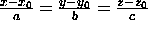These are the symmetric equations of the line. Note that we must have a, b, and c non-zero to use this representation; the line in the example above would have symmetric equations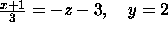#### Example

Where does the line in the previous example intersect the xy-plane? Well, the line intersects the xy-plane when z=0. From the parametric equation for z, we see that we must have 0=-3-t which implies t=-3. Thus, x=-1+3t=-10 and y=2. The line intersect the xy-plane at the point (-10,2).

#### Planes

A plane is determined by a point P_0 in the plane and a vector n (called the normal vector) orthogonal to the plane. If P is any other point in the plane and r_0 and r are the position vectors of the points P_0 and P, respectively, then an equation of the plane is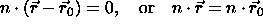since each vector in the plane must be orthogonal to the normal vector n and the vector r-r_0 is a vector in the plane. Either of these equations is called a vector equation of the plane. With the substitutions n=<a,b,c>, r=<x,y,z>, and r_0=<x_0,y_0,z_0> and some simplification we have an equation of the plane passing through the point P_0(x_0,y_0,z_0) with normal vector <a,b,c>: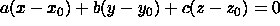This is frequently written as ax+by+cz=d. Notice that the normal vector can be identified directly from the coefficients on the left hand side of the equation: n=<a,b,c>. As with equations of lines in three dimensions, it should be noted that there is not a unique equation for a given plane. The graph of the plane -2x-3y+z=2 is shown with its normal vector.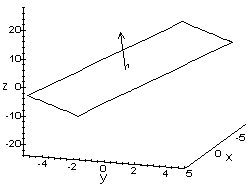#### Example

Find an equation of the plane passing through the points P(1,-1,3), Q(4,1,-2), and R(-1,-1,1). Since we are not given a normal vector, we must find one. By taking the cross product of the vector a from P to Q and the vector b from Q to R, we obtain a vector which is orthogonal to each of the original vectors (and thus orthogonal to the plane). Hence, a=<3,2,-5>, b=<-5,-2,3>, and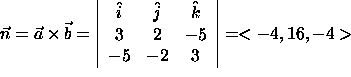So, an equation of the plane is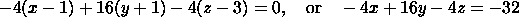Correction The normal vector and the equation of the plane above above are incorrect. The correct normal vector is <-4,16,4>. The correct equation is:

-4(x-1)+16(y+1)+4(z-3)=0 which implies -4x+16y+4z=-8

Two planes are parallel if their normal vectors are parallel (constant multiples of one another). It is easy to recognize parallel planes written in the form ax+by+cz=d since a quick comparison of the normal vectors n=<a,b,c> can be made.

#### Example

Find the formula for the distance D from a point P_0(x_0,y_0,z_0) to the plane ax+by+cz+d=0. Let P_1(x_1,y_1,z_1) be any other point in the plane. Let r =<x_0-x_1,y_0-y_1,z_0-z_1> be the vector from P_1 to P_0. Then, from the figure above, the distance D from the point to the plane is the scalar projection of the vector r onto the normal vector n: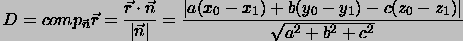But, since the point P_1 lies in the plane, -ax_1-by_1-cz_1=d. Hence, the distance D from the point P_0 to the plane is